How to Calculate and Solve for the Average Pressure of a Reservoir Fluid Flow | The Calculator Encyclopedia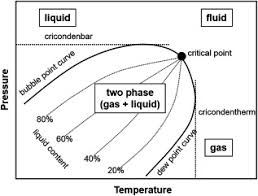The above image represents the reservoir fluid.

To compute for the reservoir fluid, two essential parameters are needed and these parameters are External pressure (Peand Flowing Bottom-Hole Pressure (Pwf).

The formula for calculating the average pressure of the reservoir fluid:

P* = √[Pe² + Pwf² / 2]

Where;

P* = Average Pressure
Pe = External Pressure
Pwf = Flowing Bottom-Hole Pressure

Let’s solve an example;
Find the average pressure of a reservoir fluid with an external pressure of 19 and Flowing bottom-hole pressure is 15.

This implies that;

Pe = External Pressure = 19
Pwf = Flowing Bottom-Hole Pressure = 15

P* = √[Pe² + Pwf² / 2]
P* = √[19² + 15² / 2]
P* = √[361 + 225 / 2]
P* = √[586 / 2]
P* = √
P* = 17.117

Therefore, the average pressure is 17.117 psi;.

Calculating the External Pressure when the Average Pressure and the Flowing Button-Hole Pressure is Given.

Pe = √[[P* x 2]² – Pwf²]

Where;

Pe = External Pressure
P* = Average Pressure
Pwf = Flowing Bottom-Hole Pressure

Let’s solve an example;
Find the external pressure of a reservoir fluid with an average pressure of 24 and Flowing bottom-hole pressure is 16.

This implies that;

P* = Average Pressure = 24
Pwf = Flowing Bottom-Hole Pressure = 16

Pe = √[[P* x 2]² – Pwf²]
Pe = √[[24 x 2]² – 16²]
Pe = √
Pe = 45.25

Therefore, the external pressure is 45.25.

Calculating the Flowing Button-Hole Pressure when the Average Pressure and the External Pressure is Given.

Pwf = √[[P* x 2]² – Pe²]

Where;

Pwf = Flowing Bottom-Hole Pressure
Pe = External Pressure
P* = Average Pressure

Let’s solve an example;
Find the Flowing bottom-hole pressure of a reservoir fluid with an average pressure of 34 and external pressure is 17.

This implies that;

P* = Average Pressure = 34
Pe = External Pressure = 17

Pwf = √[[P* x 2]² – Pe²]
Pwf = √[[34 x 2]² – 17²]
Pwf = √[4624 – 289]
Pwf = √
Pwf = 65.84

Therefore, the flowing button-hole pressure is 65.84.

Nickzom Calculator – The Calculator Encyclopedia is capable of calculating the average pressure.

To get the answer and workings of the average pressure using the Nickzom Calculator – The Calculator Encyclopedia. First, you need to obtain the app.

You can get this app via any of these means:

To get access to the professional version via web, you need to register and subscribe for NGN 1,500 per annum to have utter access to all functionalities.
You can also try the demo version via https://www.nickzom.org/calculator

Android (Paid) – https://play.google.com/store/apps/details?id=org.nickzom.nickzomcalculator
Android (Free) – https://play.google.com/store/apps/details?id=com.nickzom.nickzomcalculator
Apple (Paid) – https://itunes.apple.com/us/app/nickzom-calculator/id1331162702?mt=8
Once, you have obtained the calculator encyclopedia app, proceed to the Calculator Map, then click on Petroleum under EngineeringNow, Click on Reservoir Fluid Flow under Petroleum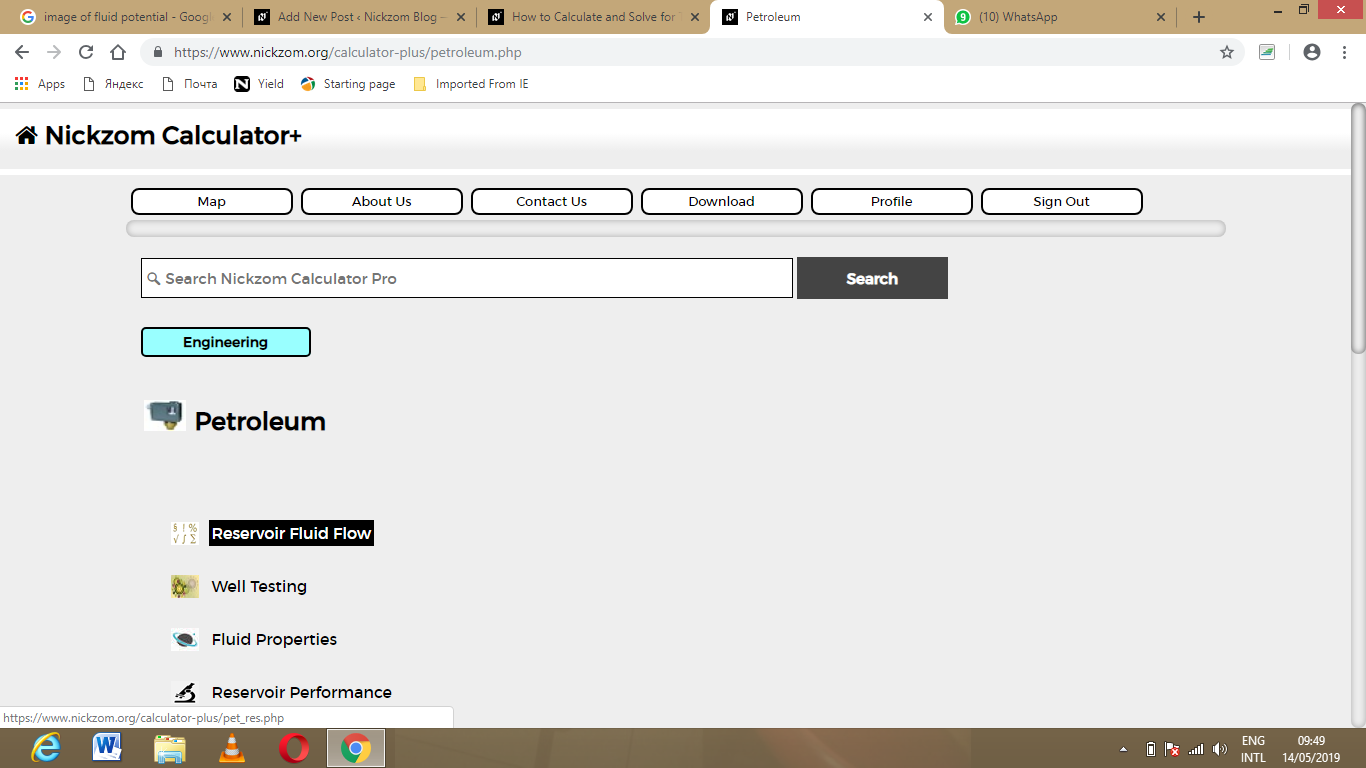Now, Click on Average Pressure under Reservoir Fluid Flow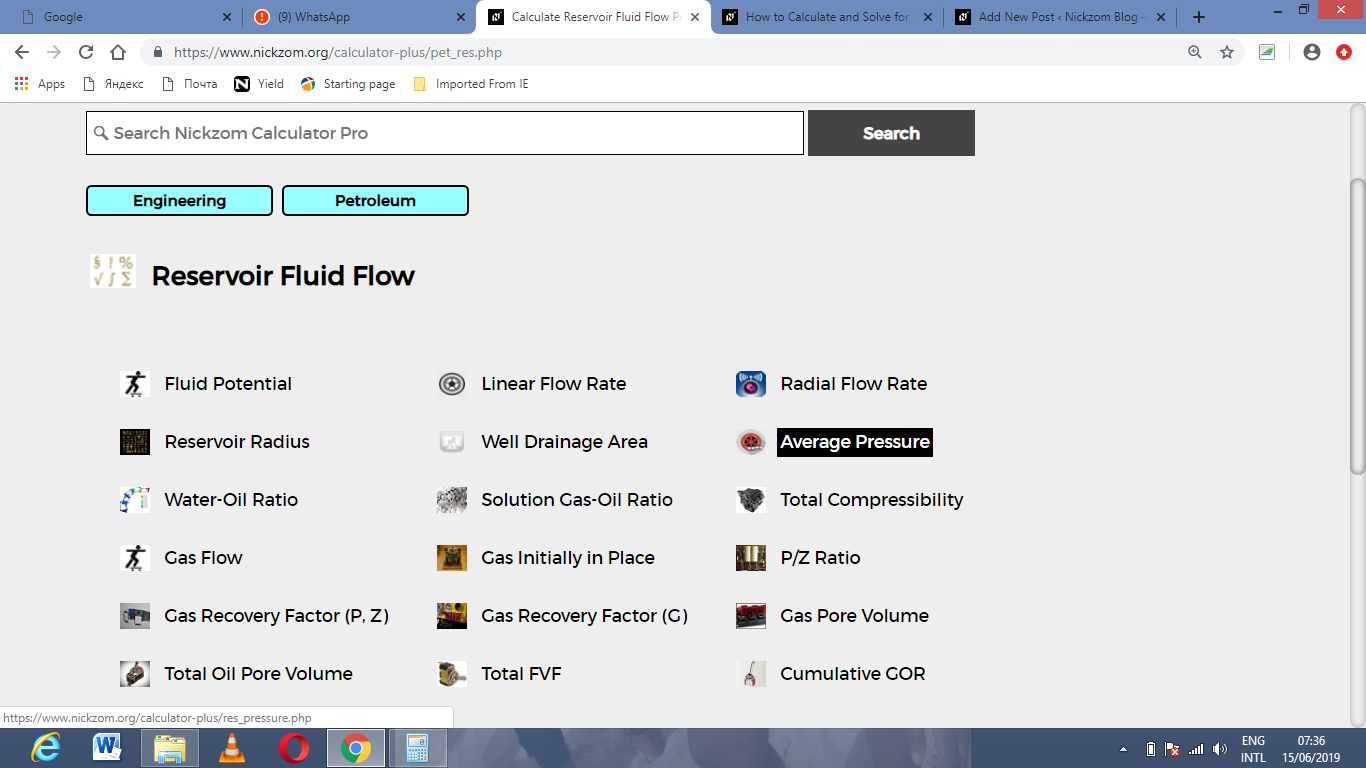The screenshot below displays the page or activity to enter your value, to get the answer for the average pressure according to the respective parameter which are the External pressure (Peand Flowing Bottom-Hole Pressure (Pwf).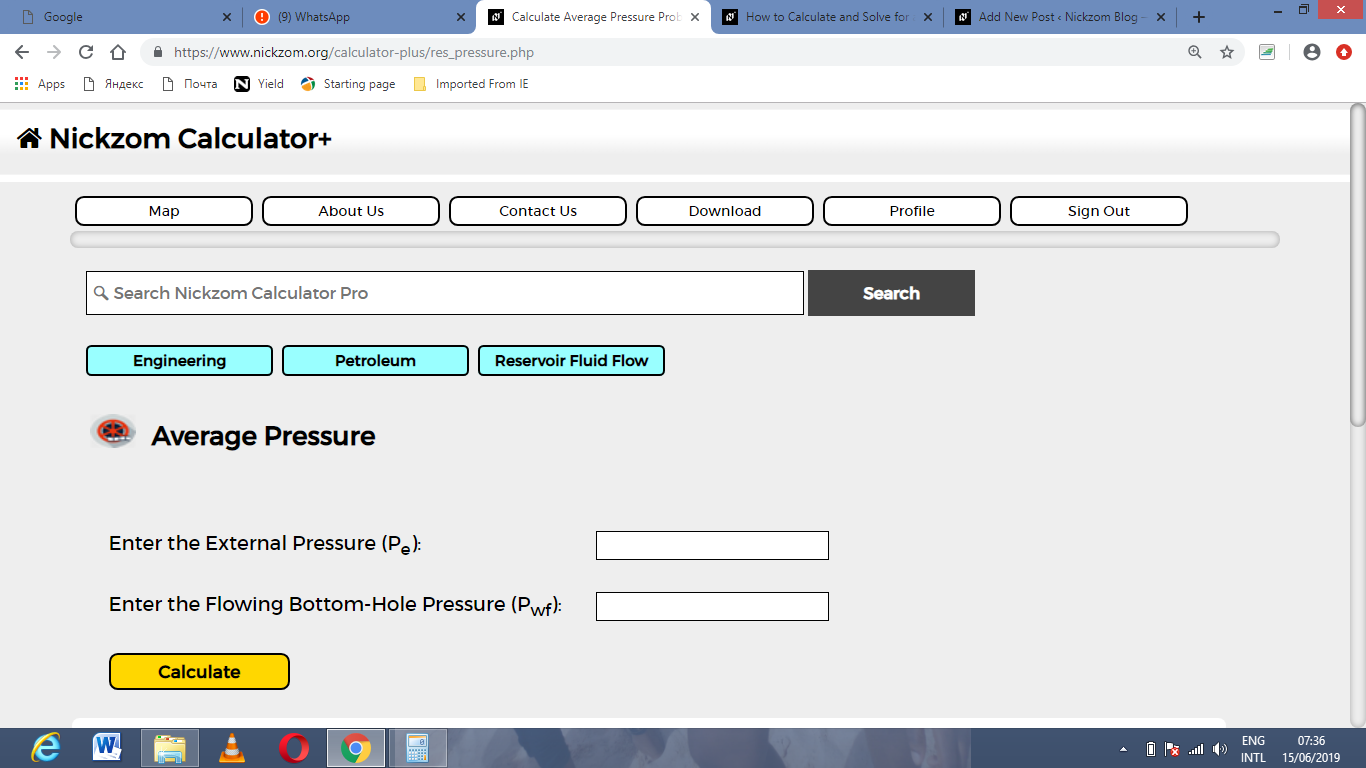Now, enter the value appropriately and accordingly for the parameter as required by the External pressure (Pe) is 19 and Flowing Bottom-Hole Pressure (Pwfis 15.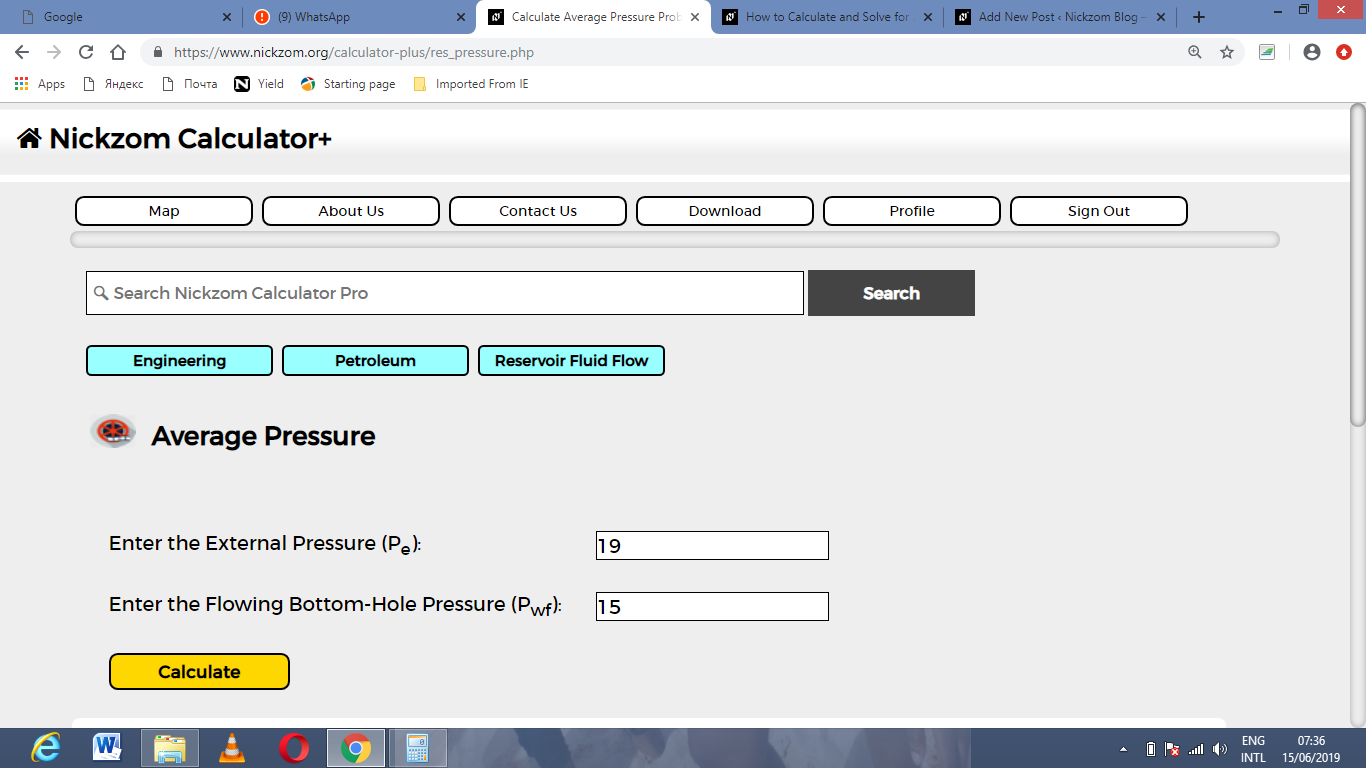Finally, Click on Calculate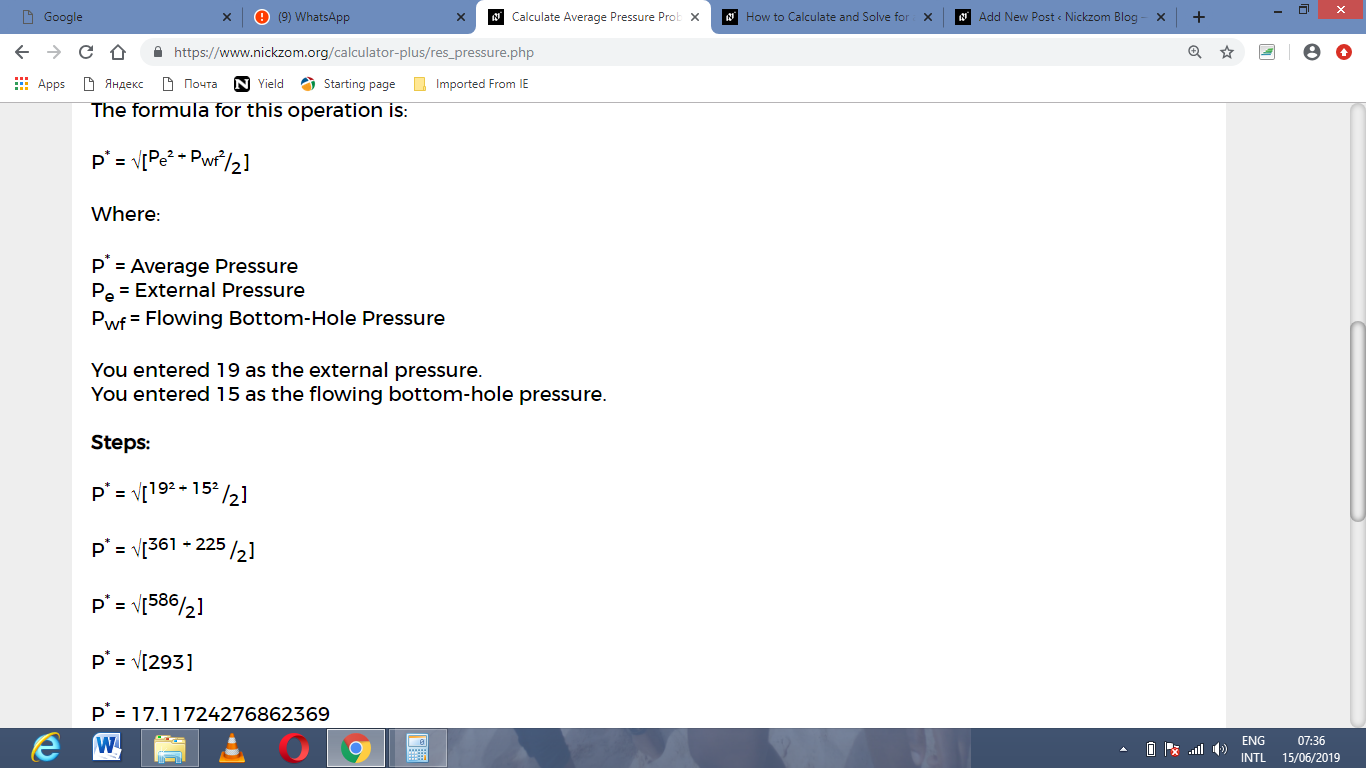As you can see from the screenshot above, Nickzom Calculator– The Calculator Encyclopedia solves for the average pressure and presents the formula, workings and steps too.